Visitors Online: 0 | Saturday 14th December 2019CBSE Guess > Papers > Important Questions > Class XII > 2008 > Maths > Maths By Mrs. Geethanjali Bhatt CBSE CLASS XII
Q. 1. Let f:R -> Range of f given by f(x)= (x+1)2 - 1 and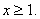The set S = {x: f(x) = f -1(x)} is {0, -1} {0, 1} {-1, 1} {1, 1}. Q. 2. The minimum number of elements that must be added to the relation R = { (1,2), (2,3) } on the set of natural numbers so that it is an equivalence is 4 7 6 5 Q. 3. Let ‘R’ be a reflexive relation on a finite set ‘A’ having ‘n’ elements and let there be ‘m’ ordered pairs in ‘R’. Then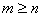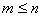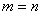none of these. Q. 4. Let ‘X’ be a family of sets and ‘R’ be a relation defined by ‘A is disjoint from B’ .Then R is Reflexive Symmetric Anti symmetric Transitive Q. 5. The number of surjections from A = {1, 2, 3, ….. n} on to B = {a, b} is nP2 2n - 2 2n - 1 none Q. 6. If a function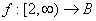defined by f(x) = x2 - 4x + 5 is a bijection, then B = R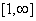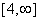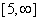Q. 7. Let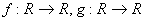be two functions given by f(x) = 2x - 3, g (x) = x3 + 5. fog-1(x) is equal to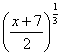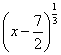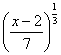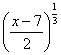Q . 8. If A = { a, b} then the number of binary operations that can be defined on A is 4 2 16 1 Q. 9. Let f(x) = [x] and g(x) = x - [x] then which of the following function is the zero function (f+g) (x) (fg) (x) (f-g) (x) fog (x) Q. 10. Let ‘A’ be a nonempty set and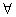be a binary operator on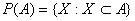defined by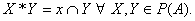Identity element of the operator * is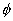A P(A) none Q. 11.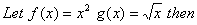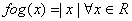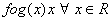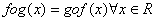none Q. 12. Let f : R --> R be the signum function. g: R -> R g(x) = [x]. fog and gof coincides on [0, 1]. True/False?